Turnerj There's so much room for activities!

# Levenshtein Distance (Part 1: What is it?)

Feb 7, 2020

The Levenshtein Distance is a deceptively simple algorithm - by looping over two strings, it can provide the "distance" (the number of differences) between the two. These differences are calculated in terms of "inserts", "deletions" and "substitutions".

The "distance" is effectively how similar two strings are. A distance of `0` would mean the strings are equal (no differences). A distance can be as high as there are as many characters in the longest string - this would mean there is absolutely nothing "similar" between these strings.

An application of the Levenshtein Distance algorithm is spell checking - knowing how similar two words are allows it to provide suggestions on what you may have intended to type.

## Calculating the Levenshtein Distance

Take the words "Saturday" and "Sunday". What do we know about these words?

• Saturday is 8 characters long and Sunday is 6.
• Relative to the beginning of the string, the shared "u" is in a different position.
• This just leaves "a", "t" and "r" from Saturday and "n" from Sunday as the only other differences.

Calculating the Levenshtein Distance of these two strings is effectively like building a matrix of values which represent the various inserts, deletions and substitutions required.

Let's build a matrix of this ourselves. We're going to start with the top row and left most column filled with numbers from `0` to each of the string's lengths.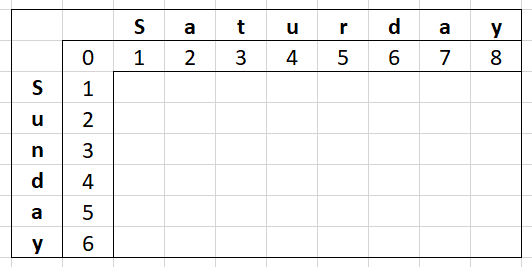Starting from the position of where the asterisks is, we will look at the cell above (the deletion cost), the cell to the left (the insertion cost) and the cell diagonally top-left (the substitution cost).

The operations to fill our cell are as follows:

• Get the minimum of the insert cost (`1`) and deletion cost (`1`), adding `1` to the result (`MIN(1,1) + 1 = 2`)
• Get the substitution cost (`0`), adding `1` to it if the letter on this column ("S") and row ("S") are different (`0 + 0 = 0`)
• Take the minimum of those numbers (`MIN(2,0) = 0`) and put that in our cell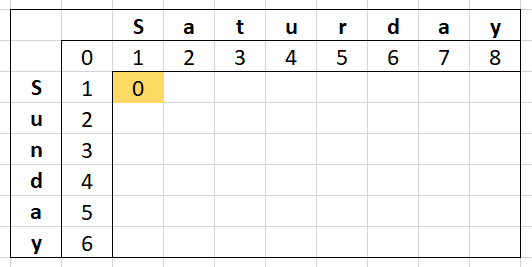Rinse and repeat for the next cell in the row:

• Get the minimum of the insert cost (`0`) and deletion cost (`2`), adding `1` to the result (`MIN(0,2) + 1 = 1`)
• Get the substitution cost (`1`), adding `1` to it if the letter on this column ("a") and row ("S") are different (`1 + 1 = 2`)
• Take the minimum of those numbers (`MIN(1,2) = 1`) and put that in our cell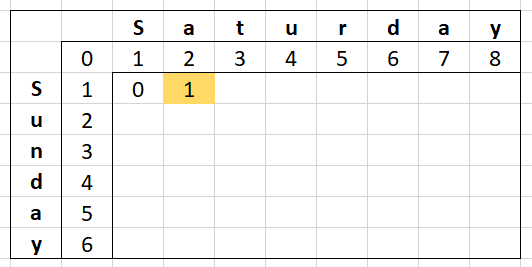This keeps going for the whole row: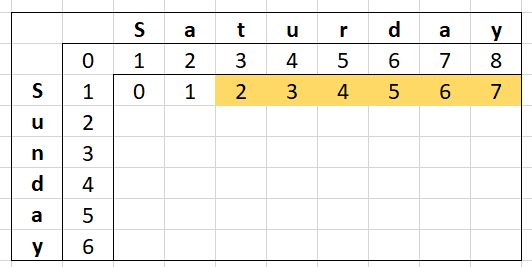And eventually the whole table: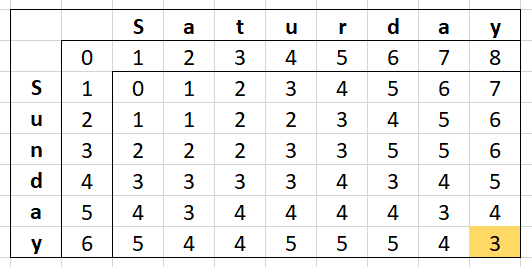The actual Levenshtein Distance can be found in the bottom-right cell (`3`). Amazing right?

## "Deceptively Simple"

I said early this algorithm is deceptively simple - I say this because of its computational complexity. This has a "Big-O" notation of `O(n*m)` where `n` is the length of one string and `m` is the length of the other. Memory-wise, building that matrix above is effectively `(n+1)*(m+1)` cells.

For the example above, there are 48 character comparisons with 96 checks for minimum value. Representing that table in memory (`(6+1)*(8+1)` cells by 4 bytes a cell, assuming 32-bit numbers) would account for 252 bytes!

Yes, I'm sure you're rolling your eyes that I'm squawking at 252 bytes. The problem is when you want to compare bigger strings. How about comparing two 1000 character strings? You'd be looking at... 4 megabytes.

That might not sound like a lot for a single lookup but now imagine doing that dozens or hundreds of times in a language that utilises garbage collection - the allocations would be huge!

When I first learnt about Levenshtein Distance I wanted to use it on web pages, to see how different two web pages are. Web pages can be quite large when counting every character in the HTML (this post as on The Practical DEV - at the time of writing - is north of 77K characters) or even just every text node (6K+ characters on this page at time of writing). Punching that into the same calculations above would be ~34 megabytes for 6K characters or a whopping 5 gigabytes for 77K characters!

Now what if we could reduce the number of cells we need from `(n+1)*(m+1)` to `(MIN(n,m)+1)*2` or even just `MIN(n,m)`? In Part 2, I'll talk about the strategies of making this algorithm faster and more memory efficient.